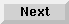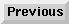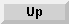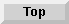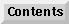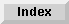5 Operators and Functions

## Arithmetic Operators

The arithmetic operators are listed in the following table:

Columns
Operators Operations Examples
-subtract10-5 = 5
-negate-2
*multiply10*5 = 50
/floating point divide15/10 = 1.5
^ or **exponentiate8^3 = 512
DIVinteger divide: A DIV B =15DIV10 = 1
SGN (A/B)*INT(ABS(A/B))-15DIV10 = -1
MODmodulo:38MOD6 = 2
A MOD B = A-(B*INT(A/B))-13MOD2 = 1
-13MOD-2 = -1

Note that, unlike algebraic notation, implied multiplication does not exist in Eloquence; thus, A times B must be written as A*B rather than just AB. The operation of raising a number to a power also requires an explicit operator; thus, AB is written as either A^B or A**B.

There are two division operators--/ and DIV. Division with the / operator (called floating-point division) results in a value in the real-precision range. When the DIV operator is used (integer division), the result is computed using the integer value of each operator. In either case, the results are returned in real precision. For example:

```3/2 = 1.5
3 DIV 2 = 1

-10/5 = -2.0
-10 DIV 5 = -2

9.999999999/1 = 9.999999999
9.999999999 DIV 1 = 9
```
The function INT(X), which is used to calculate A MOD B, returns the greatest integer less than or equal to X. So, using the formula

A MOD B = A - B * INT (A/B), we have:

```38MOD6    = 38 - 6 * INT (38/6)
= 38 - 6 * 6
= 38 - 36
= 2

-13MOD2   = -13 - 2 * INT (-13/2)
= -13 - 2 * (-7)
= -13 - (-14)
= 1

-13MOD-2  = -13 - (-2) * INT (-13/-2)
= -13 - (-2) * 6
= -13 - (-12)
= -1
```
Expressions with more than two operands are evaluated according to the following hierarchy of arithmetic operators:

```
^ **            highest
* / DIV MOD
+ -            lowest
```
Note the following examples:

```
5 + 6 * 7 = 5 + 42 = 47
5 * 6 + 7 = 30 + 7 = 37
```
If operators are at the same level, the order is from left to right in the expression:

```
30 - 40 + 100 = -10 + 100 = 90
2 + 3^2 - 1 = 2 + 9 - 1 = 11 - 1 = 10
```
Parentheses can be used to override this order, as shown in the following examples:

```
30 - (40 + 100) = 30 - 140 = -110

2 + 3^(2-1) = 2 + 3^(1) = 2 + 3 = 5

5 + 6 * 7 = 5 + 42 = 47
(5 + 6) * 7 = 11 * 7 = 77

14/7*6/4 = 2*6/4 = 12/4 = 3
14/(7*6)/4 = 14/42/4 = .333.../4 = .08333...
```
When parentheses are nested, operations within the innermost pair are performed first:

```
100/((4+6)*2) = 100/(10*2) = 10/20 = 5
2*((3+4)-5)/6 = 2*(7-5)/6 = 2*2/6 = 2*2/6 = 4/6 = .6666...
```

Eloquence Language Manual - 19 DEC 2002